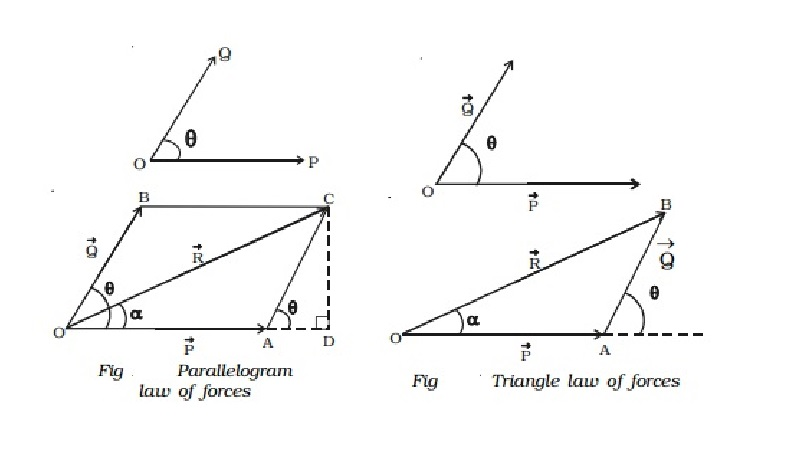Home | | Physics | | Physics | Parallelogram and Triangle law of forces

# Parallelogram and Triangle law of forcesIf two forces acting at a point are represented in magnitude and direction by the two adjacent sides of a parallelogram, then their resultant isrepresented in magnitude and direction by the diagonal passing through the point.

Parallelogram law of forces

If two forces acting at a point are represented in magnitude and direction by the two adjacent sides of a parallelogram, then their resultant isrepresented in magnitude and direction by the diagonal passing through the point.

Explanation

Consider two forces Vector P and Vector Q acting at a point O inclined at an angle θ as shown in Fig..

The forces Vector P and Vector Q are represented in magnitude and direction by the sides OA and OB of a parallelogram OACB as shown in Fig.

The resultant Vector R of the forces Vector P and Vector Q is the diagonal OC of the parallelogram. The magnitude of the resultant is

R = root[ P2 +Q2 + 2PQcos θ ]

The direction of the resultant is α = tan-1[ Qsin θ  /  P+Qcos θ ]Triangle law of forces

The resultant of two forces acting at a point can also be found by using triangle law of forces.

If two forces acting at a point are represented in magnitude and direction by the two adjacent sides of a triangle taken in order, then the closing side of the triangle taken in the reversed order represents the resultant of the forces in magnitude and direction.

Forces Vector P and Vector Q act at an angle θ. In order to find the resultant of Vector P and Vector Q, one can apply the head to tail method, to construct the triangle.

In Fig., OA and AB represent Vector P and Vector Q in magnitude and direction. The closing side OB of the triangle taken in the reversed order represents the resultant Vector R of the forces Vector P and Vector Q. The magnitude and the direction of Vector R can be found by using sine and cosine laws of triangles.

The triangle law of forces can also be stated as, if a body is in equilibrium under the action of three forces acting at a point, then the three forces can be completely represented by the three sides of a triangle taken in order.

If Vector P , Vector Q and Vector R are the three forces acting at a point and they are represented by the three sides of a triangle then P/QA =Q/AB =R/ OB.

Study Material, Lecturing Notes, Assignment, Reference, Wiki description explanation, brief detail

Related Topics